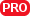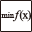#### File Exchange > Data Analysis >    Optimization SolverAuthor:
OriginLab Technical Support
9/30/2020
Last Update:
12/30/2020
84
Total Ratings:
0
File Size:
812 KB
Average Rating:File Name:
OptSolver.opx
File Version:
1.10
Minimum Versions:
Free
Summary:

Find solutions that minimize or maximize a function defined in a worksheet's cell.

Screen Shot and Video:
Description:

PURPOSE
This app can be used to find solutions that minimize or maximize a function defined in a worksheet's cell. It can also be used for curve fitting by defining the residual sum of squares as the target function for minimization.

INSTALLATION
Download the file OptSolver.opx, and then drag-and-drop onto the Origin workspace. An icon will appear in the Apps Gallery window.
NOTE: This tool requires OriginPro.

INPUT
Input for this tool includes the objective function to minimize or maximize defined in a worksheet's cell, initial values for variables in the objective function which can be placed in a column, and a column to save final solutions. If bounds are applied, two columns for lower bounds and upper bounds are required. If a variable has no lower bound or upper bound, set a missing value for that variable in columns. Note that all inputs must be in a worksheet.

The objective function in the worksheet's cell can be defined by Cell Formula or Before Formula Scripts in Set Values dialog. The Sample OPJU file in this app shows both methods: Minimize a Function example (example 1) uses Before Formula Scripts in column F, and Fit Gaussian example (example 2) uses Cell Formula in the cell G1. And Recalculate should be None in Set Values dialog, otherwise it may affect the speed.

If it is used for curve fitting, the objective function must be expression of the residual sum of squares. And a column is needed to calculate the predicted dependent variable by parameters in the column to save final solutions, and the prediction column can be evaluated by formula in Set Values dialog. Examples 2-4 in this app's Sample OPJU file demonstrate how to define it.

Formula in Set Values dialog supports LabTalk script, X-Function, OriginC function(load and compile it first) and Python function. Example 5 in this app's Sample OPJU file calls Python function in Set Values dialog:

//Before Formula Scripts
if( Python.chk("scipy")>1 )
{break 1;}

col(D)=py.frosen(col(C));

#Python Function
from scipy.optimize import rosen
import numpy as np
def frosen( p ):
x=np.array( p )
y=rosen(x)
return y

and example 3 utilizes X-Function in Set Values dialog.

//Before Formula Scripts
double dx=col(A)-col(A), y0=col(I);
conv ix:=col(D) response:=col(C) interval:=dx circular:=circular oy:=(col(E),col(F));
col(F)=y0+dx*col(F);

ALGORITHM
This tool supports three methods: Simplex, Sequential Quadratic Programming and Quadratic Approximation. NAG functions are used: nag_opt_simplex_easy, nag_opt_nlp and nag_opt_bounds_qa_no_deri. The objective function is defined in a worksheet's cell, it can have no detailed expression, and it only needs to be evaluated when variables (parameters in curve fitting) are given.

OPERATION

1. Make the worksheet for input data active. Highlight the Objective Function cell. Click the Optimization Solver icon in the Apps Gallery window to open the dialog. In the opened dialog, drag columns in the left Columns panel and drop to Column for Initial Values and Column for Solution in the right panel's Input branch. If Set Bounds in Settings branch is checked, drag and drop columns for Column for Lower Bounds and Column for Upper Bounds.
2. In the Settings branch, choose Minimum or Maximum as Target. If it is used for curve fitting, Minimum should be chosen. Select a method from Method drop-down list, three methods are available, and Simplex method is inaccessible when Set Bounds is chosen. In Options for Iteration group, set criteria for convergence, and you can choose from the drop-down list or type a value in each item.
3. In the bottom panel, click Apply button, it will run the analysis, update results in the worksheet and graph (if the graph includes the worksheet data) , and show the summary info in the dialog. The link in the summary includes detailed iteration info. If it fails, the summary info will show the failure details, and you can adjust the method or iteration options. Click Init button, and it will update results in the worksheet and graph with initial values. Click OK button, it will close the dialog, run the analysis, update results in the worksheet and graph, and show info in Messages Log. See iteration details in the app folder's log.txt file.

Sample OPJU File
This app provides a sample OPJU file. Right click on the Optimization Solver icon in the Apps Gallery window, and choose Show Samples Folder from the short-cut menu. A folder will open. Drag-and-drop the project file OptSample.opju from the folder onto Origin. The sample project contains five examples in five folders: Minimize a Function, Fit Gaussian, Fit Convolution, Global Fit and Minimize a Python Function. In each example's folder, the Notes window shows detailed steps about how to set input in the worksheet and operate in the dialog.
Note: If you wish to save the OPJU after changing, it is recommended that you save to a different folder location (e.g. User Files Folder).

Summary
This tool doesn't care what your objective function is, and you can define it in the worksheet in any way. For this reason, it supports many curve fitting types in a simple way, e.g. fit with convolution, fit with integral, global fit and so on. Moreover, it needn't provide derivatives, which is necessary in most curve fitting methods, therefore it is easy and fast as a curve fitting tool. However, this also causes a drawback in curve fitting, it can't give parameter's standard errors and some statistics results. In a word, if you just want to estimate parameters in fitting function and predict the response, this tool is a good choice.